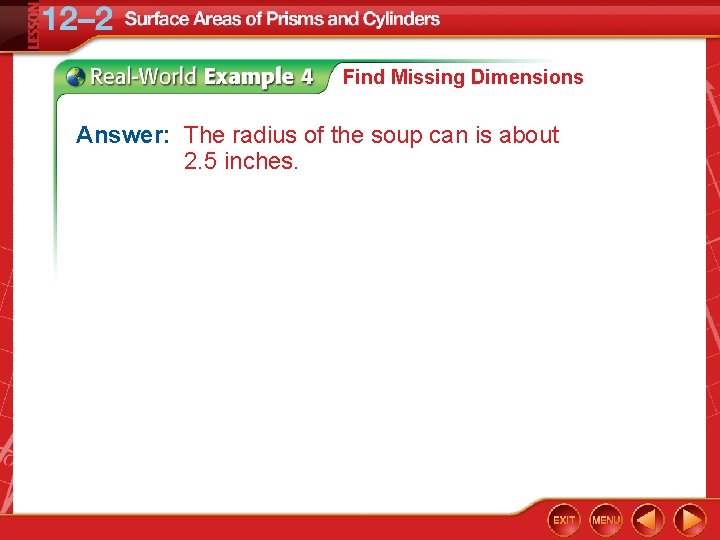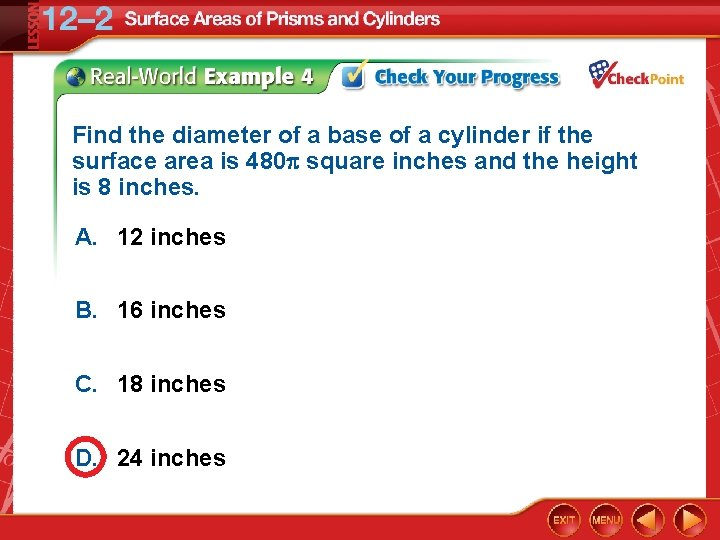# Find lateral areas and surface areas of prisms

• Slides: 21• Find lateral areas and surface areas of prisms. • Find lateral areas and surface areas of cylinders.Content Standards G. MG. 3 Apply geometric methods to solve problems (e. g. , designing an object or structure to satisfy physical constraints or minimize cost; working with typographic grid systems based on ratios). Mathematical Practices 1 Make sense of problems and persevere in solving them. 6 Attend to precision.• lateral face • lateral edge • base edge • altitude • height • lateral area • axis • composite solid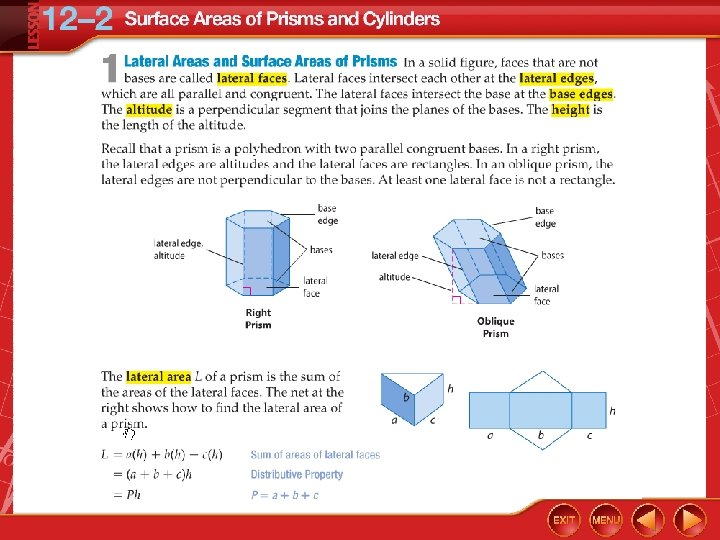Lateral Area of a Prism Find the lateral area of the regular hexagonal prism. The bases are regular hexagons. So the perimeter of one base is 6(5) or 30 centimeters. Lateral area of a prism P = 30, h = 12 Multiply. Answer: The lateral area is 360 square centimeters.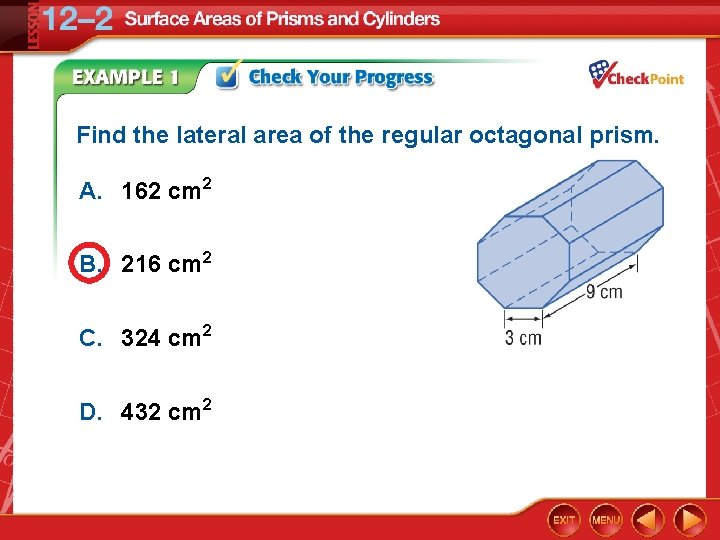Find the lateral area of the regular octagonal prism. A. 162 cm 2 B. 216 cm 2 C. 324 cm 2 D. 432 cm 2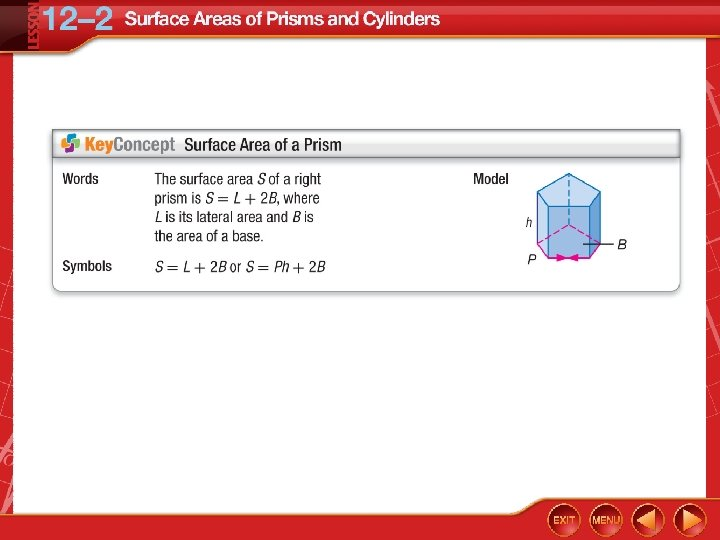Surface Area of a Prism Find the surface area of the rectangular prism.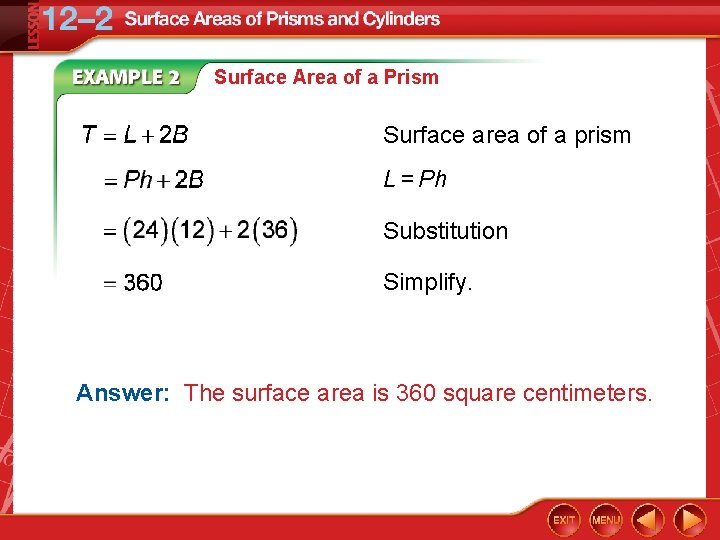Surface Area of a Prism Surface area of a prism L = Ph Substitution Simplify. Answer: The surface area is 360 square centimeters.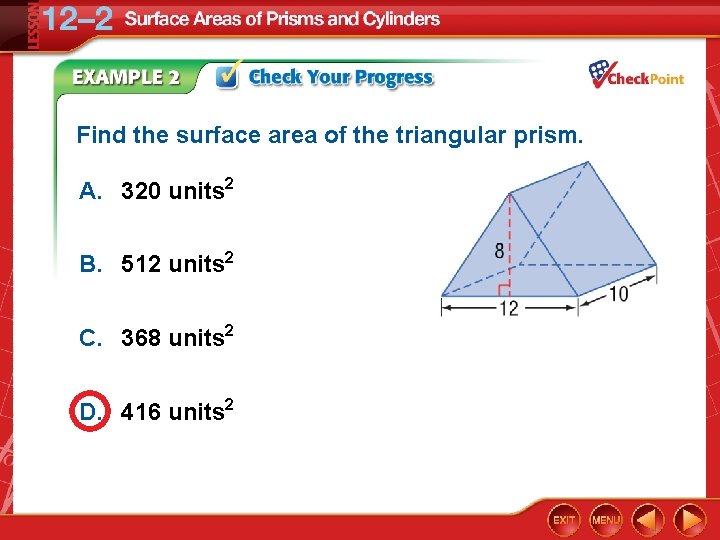Find the surface area of the triangular prism. A. 320 units 2 B. 512 units 2 C. 368 units 2 D. 416 units 2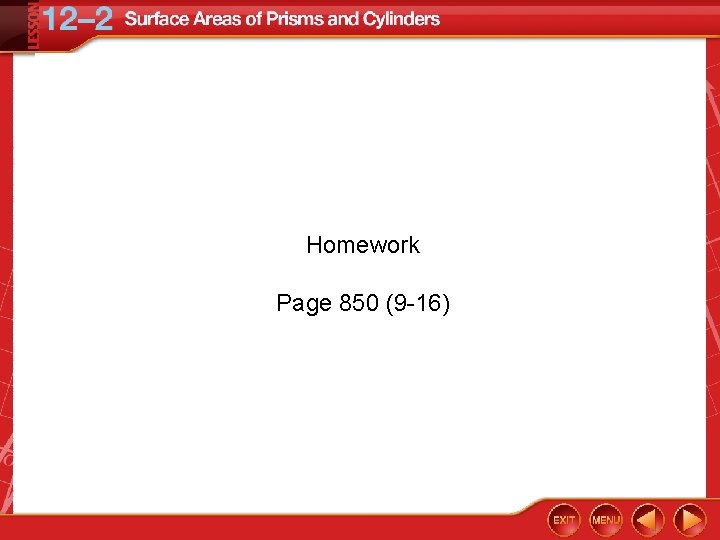Homework Page 850 (9 -16)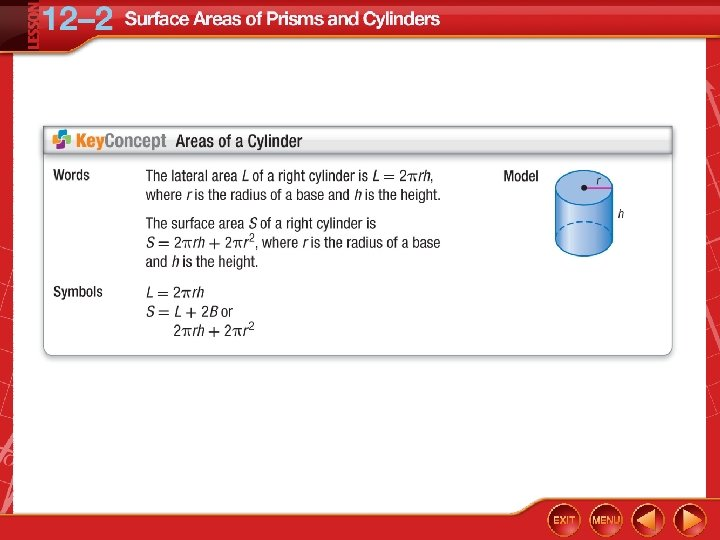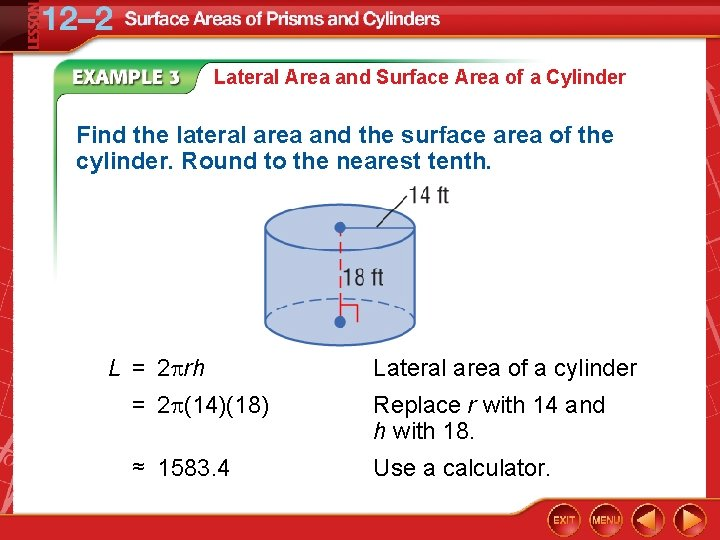Lateral Area and Surface Area of a Cylinder Find the lateral area and the surface area of the cylinder. Round to the nearest tenth. L = 2 rh Lateral area of a cylinder = 2 (14)(18) Replace r with 14 and h with 18. ≈ 1583. 4 Use a calculator.Lateral Area and Surface Area of a Cylinder S = 2 rh + 2 r 2 Surface area of a cylinder ≈ 1583. 4 + 2 (14)2 Replace 2 rh with 1583. 4 and r with 14. ≈ 2814. 9 Use a calculator. Answer: The lateral area is about 1583. 4 square feet and the surface area is about 2814. 9 square feet.Find the lateral area and the surface area of the cylinder. Round to the nearest tenth. A. lateral area ≈ 1508 ft 2 and surface area ≈ 2412. 7 ft 2 B. lateral area ≈ 1508 ft 2 and surface area ≈ 1206. 4 ft 2 C. lateral area ≈ 754 ft 2 and surface area ≈ 2412. 7 ft 2 D. lateral area ≈ 754 ft 2 and surface area ≈ 1206. 4. 7 ft 2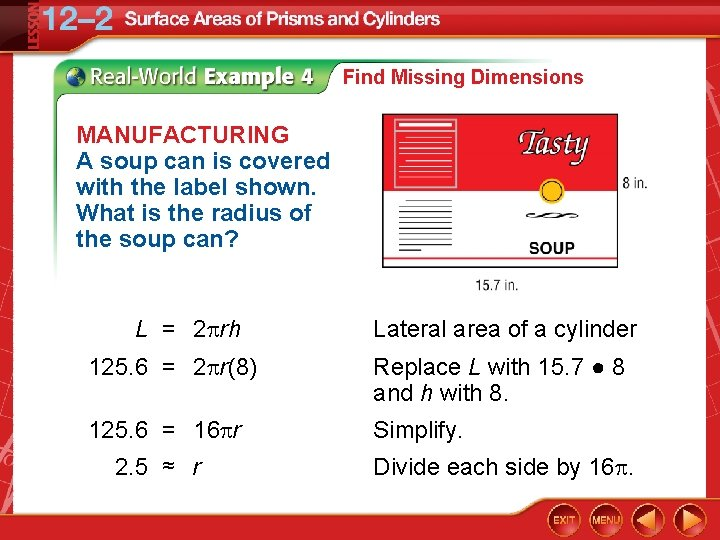Find Missing Dimensions MANUFACTURING A soup can is covered with the label shown. What is the radius of the soup can? L = 2 rh Lateral area of a cylinder 125. 6 = 2 r(8) Replace L with 15. 7 ● 8 and h with 8. 125. 6 = 16 r Simplify. 2. 5 ≈ r Divide each side by 16.